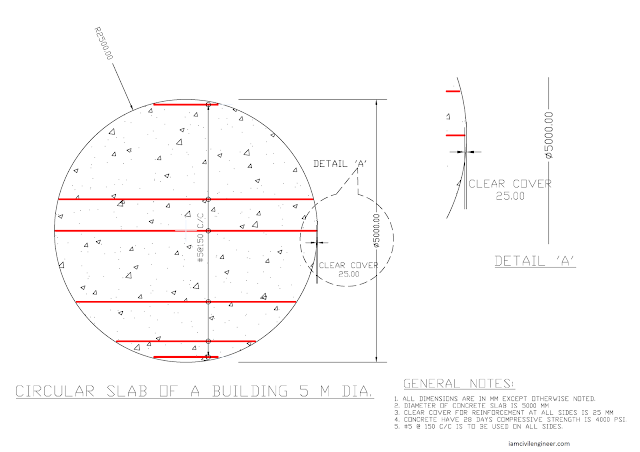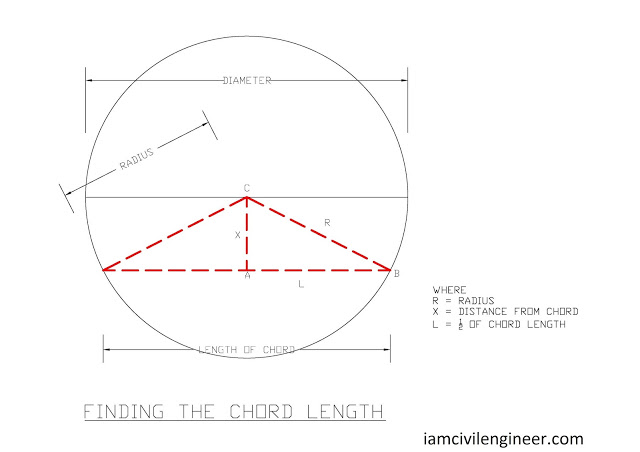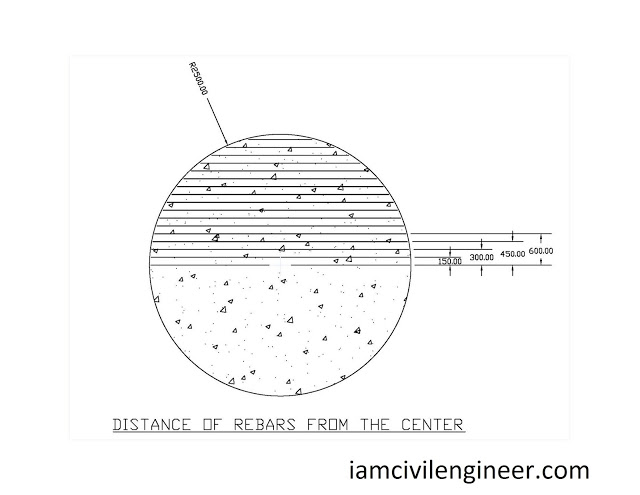# Bar Bending Schedule for Circular SlabSaad Iqbal | 🗓️Modified: March 11, 2017 | ⏳Read Time: 4 min | 👁Post Views: 18

In our previous posts we have already explained what bar bending schedule is? What information does a bar bending schedule provides? How to calculate and estimate the bar bending schedule of a simple beam.

Today in this post we will be going to establish bar bending schedule of a circular slab.

As the Bar bending Schedule for a circular slab is not that easy as it looks like; We can use AutoCAD to speed up the process; however, we cannot neglect completely the manual calculations as computer is sometimes not available in a limited resource projects.

So this post deals with both the method on how to establish bar bending schedule of a circular slab with manual calculation using Pythagoras theorem and simple triangle solutions.

So let us first go for the manual calculation of the bar bending schedule of Circular Slab:-

## By Manual CalculationGeneral Layout of Reinforcement for a Circular Slab

In the above Figure a slab is shown of 5 meters diameter which have rebar of #5 @ 150 C/C

The total diameter of the slab is 5 meters therefore the no. of rebar for the slab would come as ;-

Sometimes +1 is recommended in above equation just for ensuring sufficient numbers; however, here in order to avoid congestion it is avoided.

The arrangement of these 33 bars are shown in above figure; 1 rebar is at the center / middle of the slab while 16 rebars are upside and 16 are downside of the diagram.
The 16 bars upside and downside are identical.

### Calculation by Using Pythagoras Theorem and Solving TriangleFinding the Chord Length of a Circular Slab

We will use the arrangement as shown in above figure to calculate the length of the chord at any distance from the center. Consider Triangle ABC as shown in above figure;

Thus,

AS x which is the distance of the chord form the center is already known; thus we will solve above equation for L which is half of the chord;

To calculate the length of chord we will multiply above equation with 2;

To calculate the length of rebar we will subtract the cover from both ends which is 25 mm thus 50 mm will be subtracted; in other words the length of rebar at any distance x from the center would be ;

For our base slab; above equation would be;Distances of Reinforcement from Center of Circular Slab

For Middle Rebar

For Next rebar upside (using above equation derived)

Taking x = 150

Taking x = 300

Same procedure is adopted to get the length of all the rebars;

### Calculating Unit Weight of Rebar

For unit weight of #5 diameter rebar;

We can use the above unit weight to calculate the weight of rebar by multiplying it with length.

The final Bar bending schedule is calculated using excel sheet and is provided here;